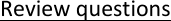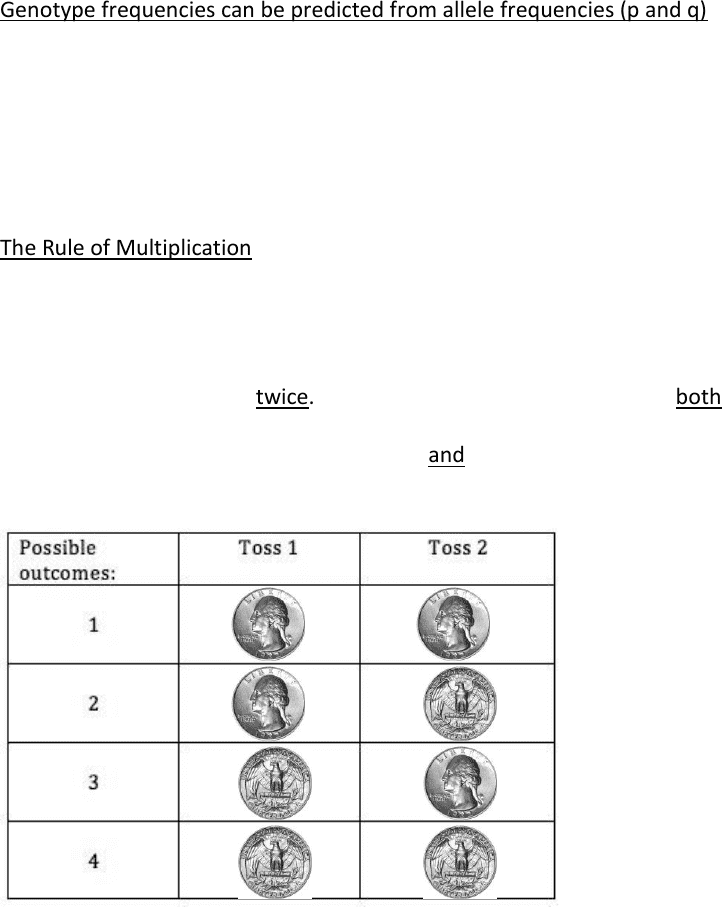Class Notes (1,100,000)
CA (650,000)
York (40,000)
NATS (3,000)
Lecture 1

# NATS 1940 Lecture Notes - Lecture 1: Genotype Frequency, Allele Frequency, Genetic Drift

Department
Natural Science
Course Code
NATS 1940
Professor
Mark Vicari
Lecture
1

This preview shows pages 1-2. to view the full 7 pages of the document.BIODIVERSITY
October 5, 2017
CHAPTER 9 POPULATION GENETICS
Review questions
1. A population consists of
25 people with the genotype AA
40 people with the genotype Aa
35 people with the genotype aa
What are the frequencies of genotypes AA, Aa, and aa, respectively?
a) 25, 40 and 35
b) 12.5, 20 and 17.5
c) 0.25, 0.40 and 0.35
d) 0.125, 0.20 and 0.175
e) 0.50, 0.80 and 0.70
25/100 =0.25
2. What is the frequency of the allele A? Of the allele a?
25 people with the genotype AA
40 people with the genotype Aa
35 people with the genotype aa
a) 0.25 and 0.35, respectively
b) 0.25 and 0.75, respectively
c) 0.65 and 0.35, respectively
d) 0.55 and 0.45, respectively
e) 0.45 and 0.55, respectively
total number of alleles: 200 (100x2)
frequency of A=p= ((25x2) + 40)/ 200 = 0.45
frequency of a = q= (40+(35x2))/200 = 0.55
check: p + q=0.45+0.55=1
3. What are the frequencies of the dominant and recessive traits (i.e. phenotypes),
respectively?
25 people with the genotype AA
40 people with the genotype Aa
35 people with the genotype aa
a) 0.65 and 0.35
b) 0.25 and 0.75

Only pages 1-2 are available for preview. Some parts have been intentionally blurred.c) 0.25 and 0.35
d) 0.40 and 0.35
e) 0.35 and 0.65
total number of phenotypes=100
frequency of dominant = (25+40)/100 = 0.65
frequency of recessive= 35/100= 0.35
check p + q=0.65+0.35=1
Genotype frequencies can be predicted from allele frequencies (p and q)
Recall: Mendel’s model of heredity states that gametes (sperm or egg) carry one of the
two alleles for each gene
Alleles segregate at random when gametes are formed (i.e., it is entirely random as to
which of two alleles a gamete receives for any given gene)
What are the odds that a sperm “picked at random”, from a random male, will carry a
dominant allele?
The Rule of Multiplication
the probability of two or more independent events occurring together is equal to the
product of their individual probabilities
Example: Tossing a coin.
o Odds of “heads” = 0.5 (or 50%)
o Toss a coin twice. What are the odds of getting heads both times?
Odds of getting heads on both the first toss and the second toss = 0.5 * 0.5 = 0.25 (or 25%)
25%, 1 in 4 chance